A few weeks ago, I introduced Boosted Configuration (neural) Networks (BCNs), with some examples of classification on toy datasets. Since then, I’ve implemented BCN for regression (continuous responses) in R, and released a Python version (built on top of the R version) of the package on PyPi. What are BCNs?

• Statistical/Machine Learning (ML) models based on combinations of single-layered feedforward neural networks ( ensembles of SLFNNs).
• Boosting algorithms possessing the Universal Approximation Property (UAP) of neural networks. Note that the UAP is only interesting if we have some ways to mitigate the overfitting/slowing down the convergence (e.g via regularization, learning rates, etc.).

One important point that I touched upon in BCNs introductory post was the time-consuming computation of weak learners’ (the SLFNNs) weights and biases, when the number of covariates is high. I implied that stats::nlminb – a good candidate for solving for weights and biases in BCN’s loop – was a derivative-free optimizer. It’s not the case. More precisely, indeed, stats::nlminb does not require input gradients or hessians. But numerical approximations of the objective function’s gradient and hessian are computed internally, if not provided (e.g because they are difficult to derive analytically).

After publishing the post, I was curious to see how stats::nlminb could behave on difficult problems, versus derivative-free optimizers. For this purpose:

• I’ve used 8 of the 23 benchmark functions extensively described in references  and  below: $$f_1, f_2, f_3, f_4, f_6, f_9, f_{10}, f_{11}$$. For the results to be relatively comparable, only functions with inputs of dimension 30, and minimum equal to 0 at (0, 0, …, 0) were considered. It’s worth mentioning that the Genetic Algorithm (GA) presented in  – actually, any GA – can’t be used for obtaining SLFNNs’ weights and biases in the BCN loop. GAs aren’t fast enough in this particular context.
• The derivative-free optimization methods (used in bcn::bcn) that I benchmarked alongside stats::nlminb (default method) are:
• dfoptim::hjkb: Hooke-Jeeves derivative-free minimization algorithm
• minqa::bobyqa: minimizes a function of many variables by a trust region method that forms quadratic models by interpolation
• bcn::randomsearch: a naive Random Search
• For each optimizer except randomsearch, different starting points are simulated 100 times, and 4 indicators are measured:
• Manhattan distance (L1-norm of the difference) between the parameter found by the optimizer and (0, 0, …, 0)
• Objective function’s value at optimal point (must be 0 if the global minimum is reached)
• Number of objective function’s evaluations in the optimizer
• Timing in seconds

Packages for the demo

suppressWarnings(library(ggplot2))
suppressWarnings(library(patchwork))
suppressMessages(library(dplyr))
suppressWarnings(library(minqa))
suppressWarnings(library(dfoptim))
suppressWarnings(library(bcn))
suppressWarnings(library(skimr))


Manhattan distance

Will be used below, to see if the minimum found by an optimizer is close to the global minimum (0, 0, …, 0). This indicator is a bit unfair to the chosen optimization methods, which are not conceived to find a global minimum. But speed is required in the BCN boosting loop, and some global optimizer could be prohibitively slow in this context.

manhattan_distance <- function(x, y)
{
sum(abs(x - y))
}


Objective functions from  and 

The file “v42i11-extra.R” containing the 23 benchmark objective functions from  and  can be downloaded from: https://www.jstatsoft.org/article/view/v042i11.

# get the 23 objective functions

set.seed(12934)

ndim <- 30

# 1, 2, 3, 4, 6, 9, 10, 11 # min at (0, 0, ..., 0) equal to 0
testsolutions <- vector("list", 13)
testsolutions[] <- rep(0, ndim)
testsolutions[] <- rep(0, ndim)
testsolutions[] <- rep(0, ndim)
testsolutions[] <- rep(0, ndim)
testsolutions[] <- rep(0, ndim)
testsolutions[] <- rep(0, ndim)
testsolutions[] <- rep(0, ndim)
testsolutions[] <- rep(0, ndim)

# 1, 2, 3, 4, 6, 9, 10, 11 # min at (0, 0, ..., 0) equal to 0
testsolutionsvalues <- vector("list", 13)
testsolutionsvalues[] <- 0
testsolutionsvalues[] <- 0
testsolutionsvalues[] <- 0
testsolutionsvalues[] <- 0
testsolutionsvalues[] <- 0
testsolutionsvalues[] <- 0
testsolutionsvalues[] <- 0
testsolutionsvalues[] <- 0


Main loop (compute the indicators)

# number of starting points/replications
reps <- 100

# number of objective functions used in the benchmarks
n_funcs <- 8

# number of optimizers
n_methods <- 4

# will contain the benchmarking results
results <- matrix(0, nrow=reps*n_funcs*n_methods,
ncol=7)
colnames(results) <- c("fn", "rep", "method",
"dist_to_sol", "value_at_sol",
"fevals", "timing")
results <- as.data.frame(results)

counter <- 1

#pb <- txtProgressBar(min = 0, max = reps*n_funcs*n_methods, style = 3)

# functions 1, 2, 3, 4, 6, 9, 10, 11 # min at (0, 0, ..., 0) equal to 0
for (i in c(1, 2, 3, 4, 6, 9, 10, 11))
{
lbounds <- testbounds[[i]][,1]
ubounds <- testbounds[[i]][,2]
testfunc <- testfuncs[[i]]

for (j in 1:reps)
{
current_seed <- j*1000 + i

set.seed(current_seed)

starting_point <- lbounds + (ubounds-lbounds)*runif(length(lbounds))

ptm <- proc.time()
obj_randomsearch <- bcn::random_search(objective = testfunc,
lower = lbounds,
upper = ubounds,
seed = current_seed,
control = list(iter.max = 10000))
timing_randomsearch <- proc.time() - ptm

ptm <- proc.time()
obj_bobyqa <- minqa::bobyqa(par = starting_point,
fn = testfunc,
lower=lbounds,
upper=ubounds,
control = list(maxfun = 10000))
timing_bobyqa <- proc.time() - ptm

ptm <- proc.time()
obj_nlminb <- stats::nlminb(start = starting_point,
objective = testfunc,
lower=lbounds,
upper=ubounds,
control = list(eval.max = 10000))
timing_nlminb <- proc.time() - ptm

ptm <- proc.time()
obj_hjkb <- suppressWarnings(dfoptim::hjkb(par = starting_point,
fn = testfunc,
lower=lbounds,
upper=ubounds,
control = list(maxfeval = 10000)))
timing_hjkb <- proc.time() - ptm

for (k in c('randomsearch', 'bobyqa', 'nlminb', 'hjkb'))
{
results[counter, "fn"] <- paste0("f", i)
results[counter, "rep"] <- j
results[counter, "method"] <- k

# distance to solution
if (k == "randomsearch")
{
results[counter, "dist_to_sol"] <- manhattan_distance(obj_randomsearch$par, testsolutions[[i]]) results[counter, "value_at_sol"] <- obj_randomsearch$objective
results[counter, "fevals"] <- obj_randomsearch$iterations results[counter, "timing"] <- timing_randomsearch } if (k == "bobyqa"){ results[counter, "dist_to_sol"] <- manhattan_distance(obj_bobyqa$par, testsolutions[[i]])
results[counter, "value_at_sol"] <- obj_bobyqa$fval results[counter, "fevals"] <- obj_bobyqa$feval
results[counter, "timing"] <- timing_bobyqa
}

if (k == "nlminb"){
results[counter, "dist_to_sol"] <- manhattan_distance(obj_nlminb$par, testsolutions[[i]]) results[counter, "value_at_sol"] <- obj_nlminb$objective
results[counter, "fevals"] <- as.integer(obj_nlminb$evaluations) results[counter, "timing"] <- timing_nlminb } if (k == "hjkb"){ results[counter, "dist_to_sol"] <- manhattan_distance(obj_hjkb$par, testsolutions[[i]])
results[counter, "value_at_sol"] <- obj_hjkb$value results[counter, "fevals"] <- obj_hjkb$feval
results[counter, "timing"] <- timing_hjkb
}

#setTxtProgressBar(pb, counter)
counter <- counter + 1
}

}
}
#close(pb)

results$log_value_at_sol <- log(results$value_at_sol)
results$log_fevals <- log(results$fevals)


Print random rows in results

print(results[sample.int(n = nrow(results), size = 20), 1:7])


Summary of results

results %>%
group_by(method) %>%
summarise(median_dist_to_sol = median(dist_to_sol),
median_value_at_sol = median(value_at_sol),
median_feval = median(log_fevals),
median_timing = median(timing))


Detailed summary (boxplots) for each objective function

Summary for $$f_{1}$$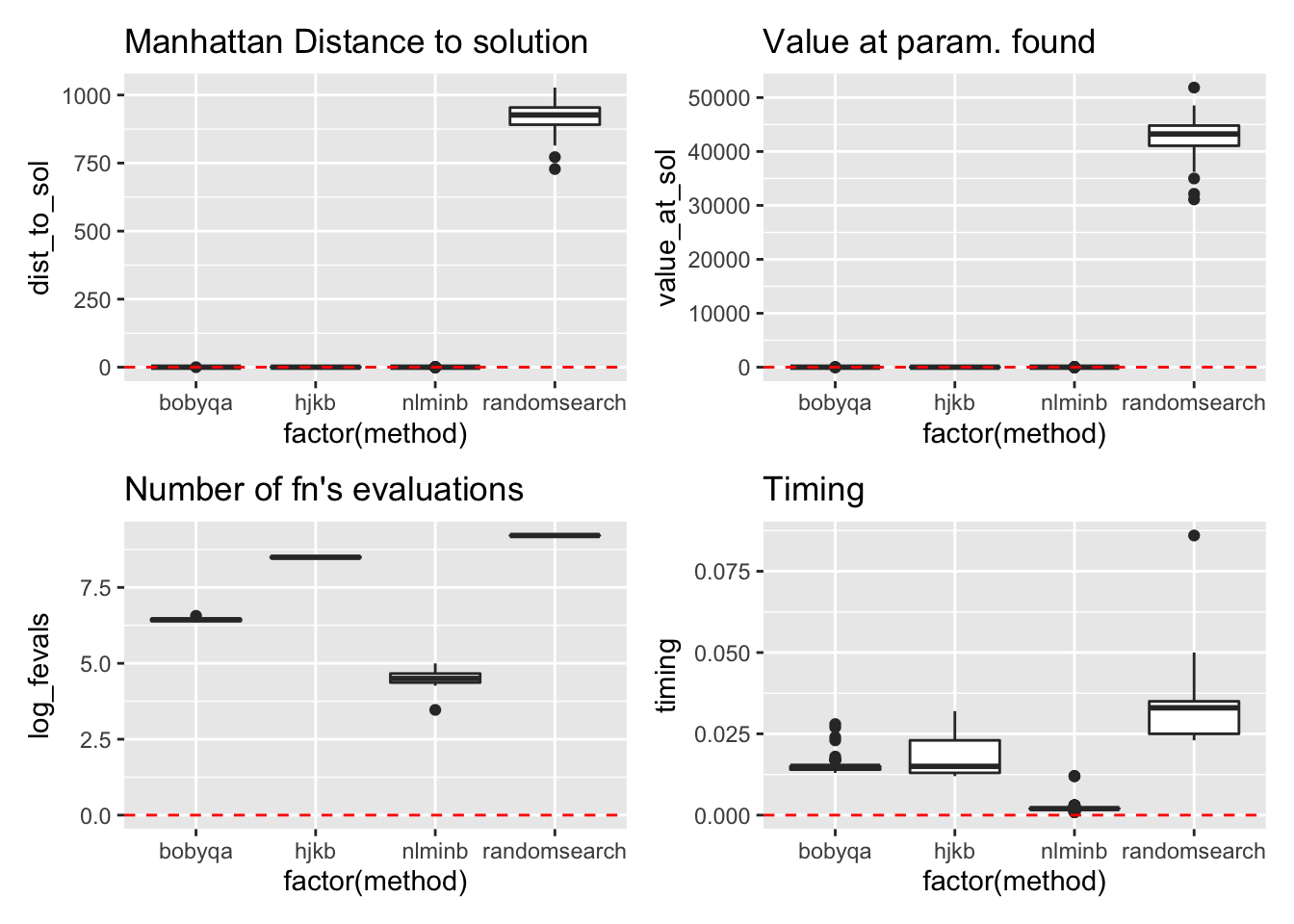Summary for $$f_{2}$$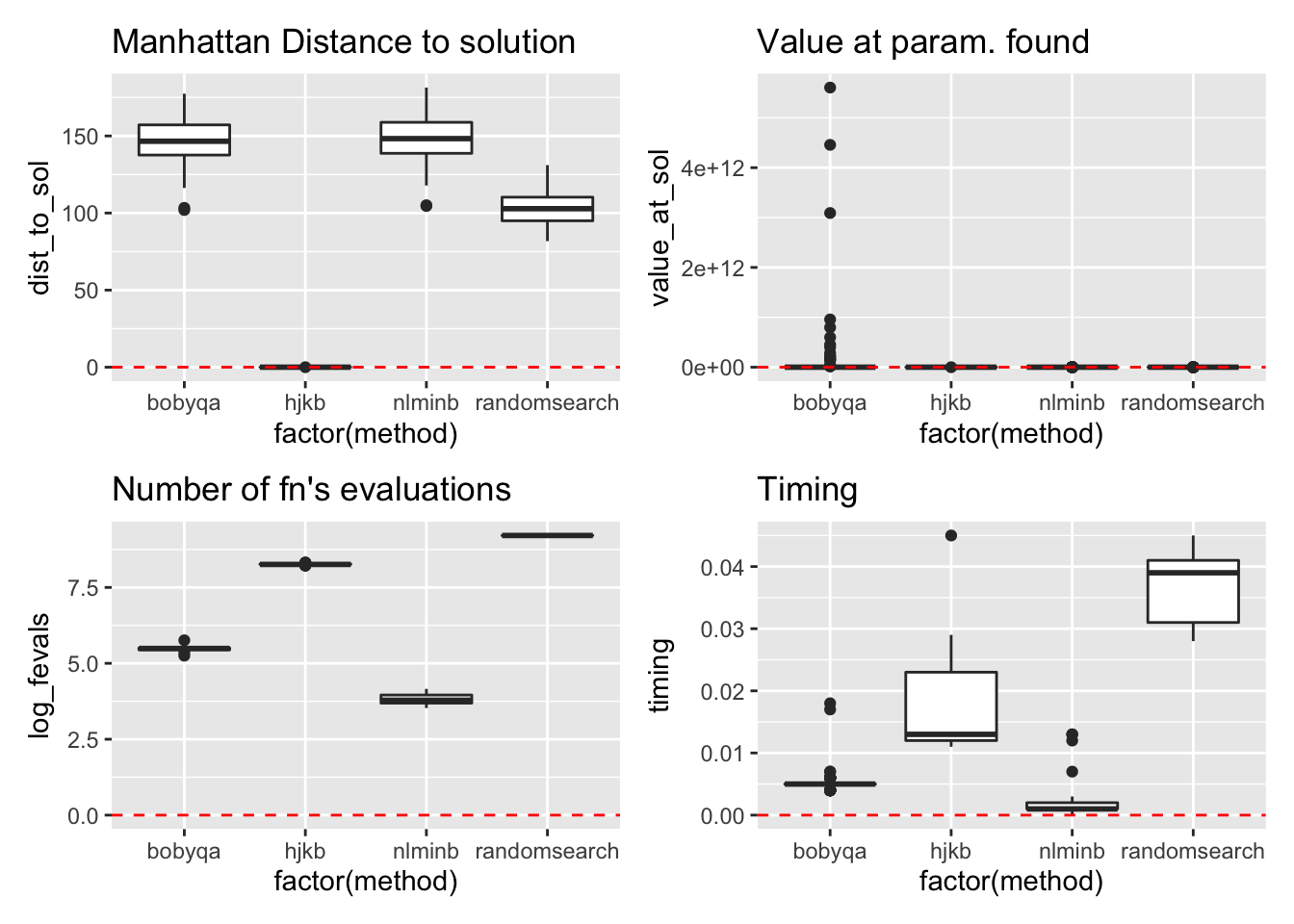Summary for $$f_{3}$$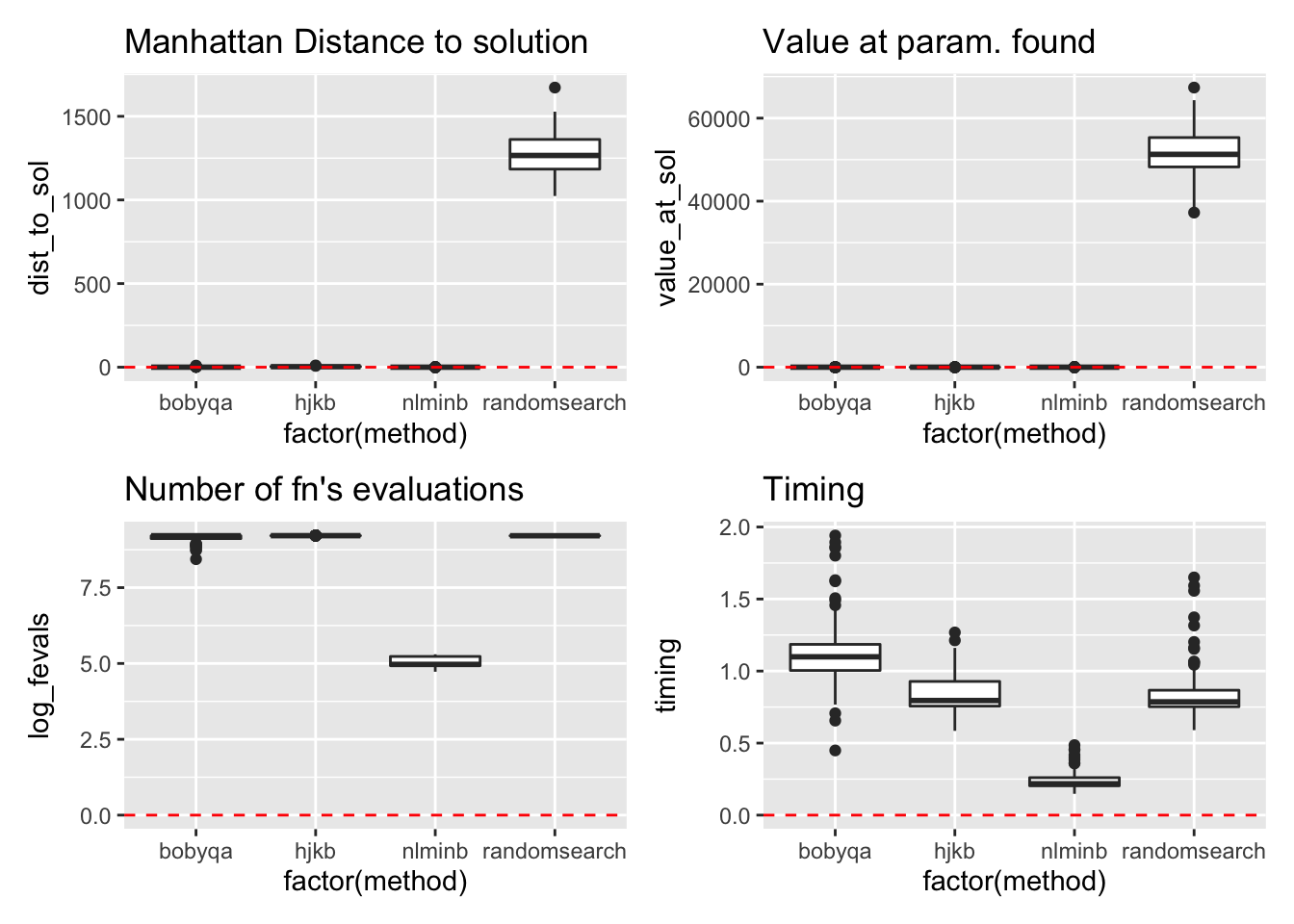Summary for $$f_{4}$$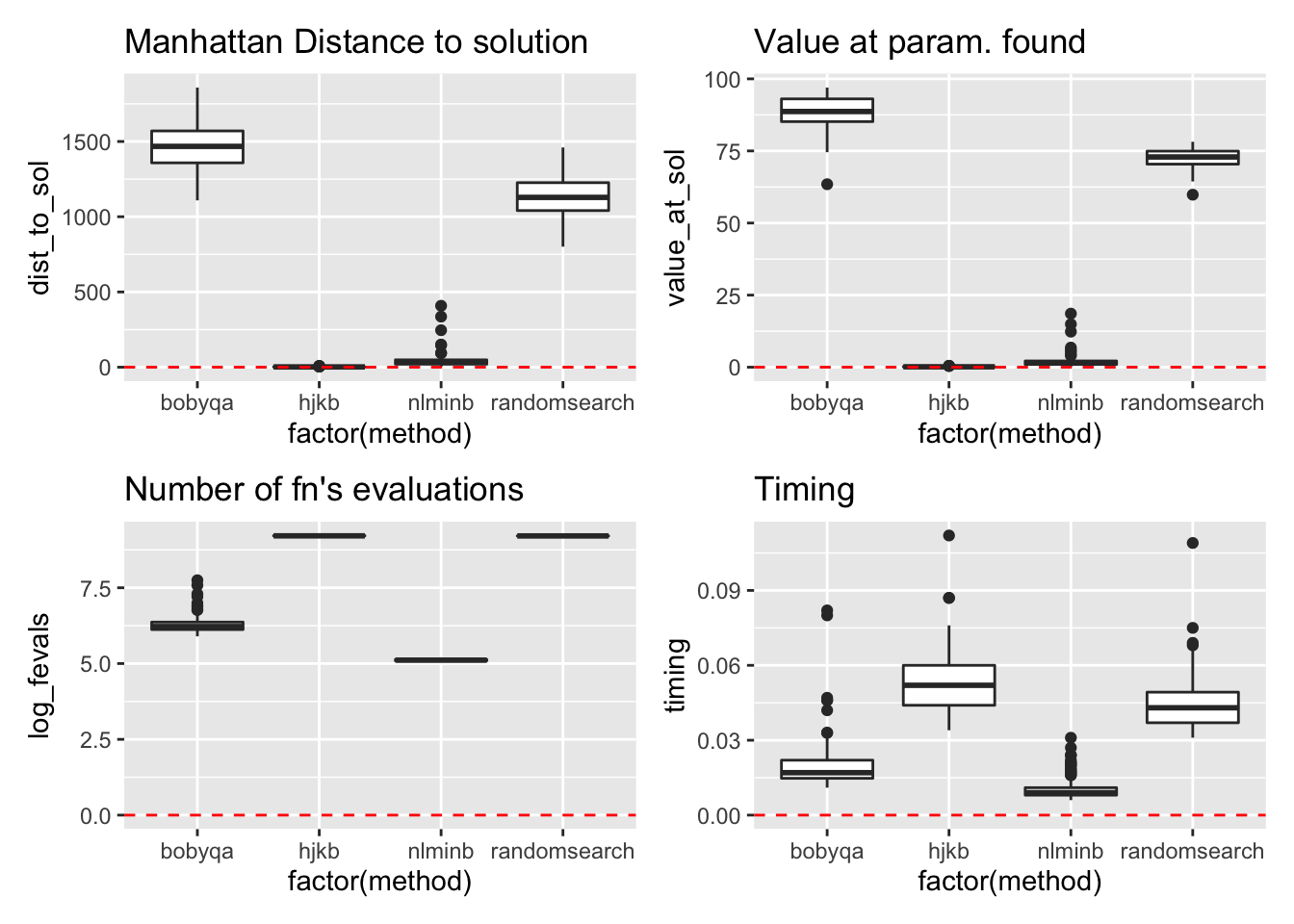Summary for $$f_{6}$$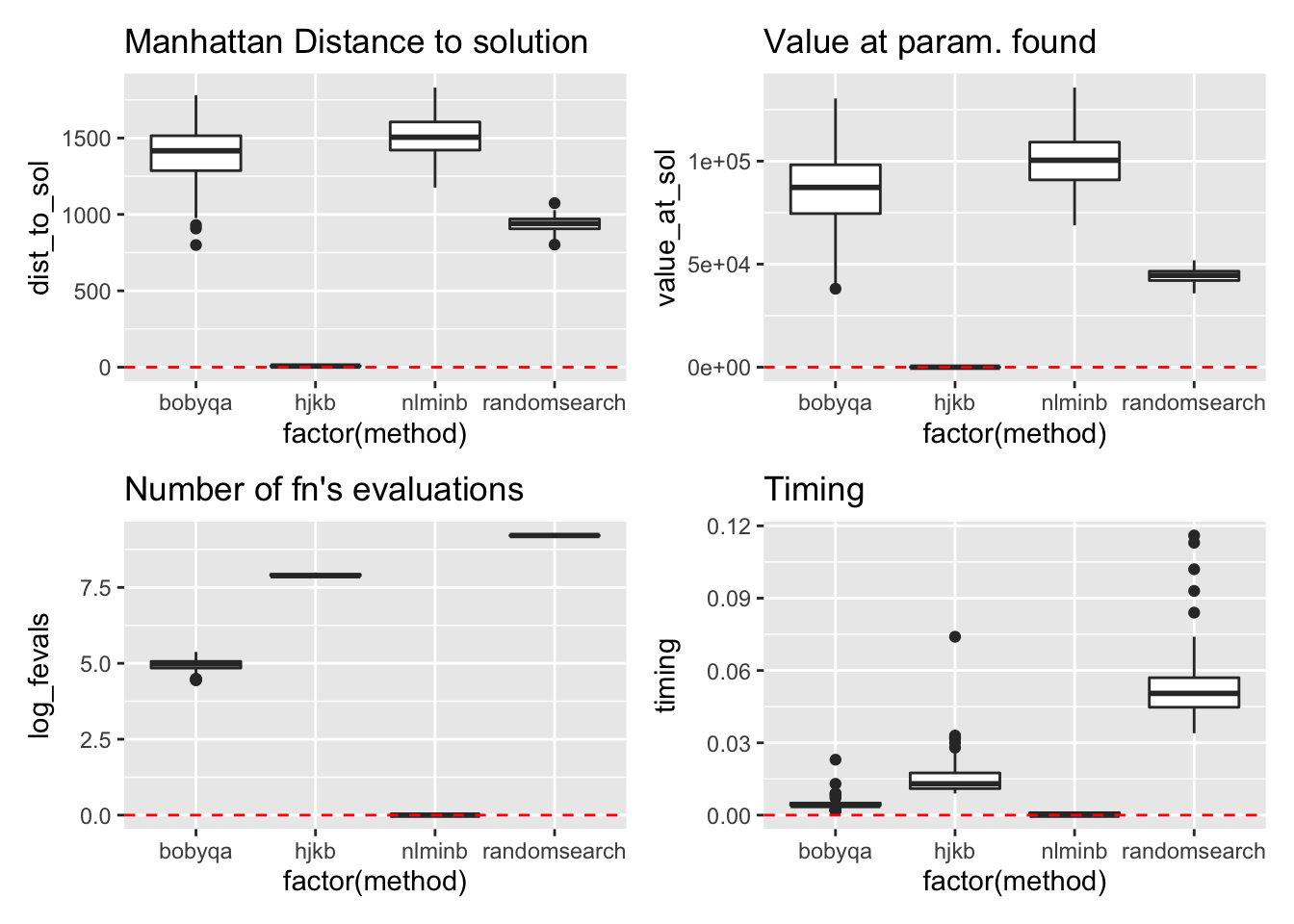Summary for $$f_{9}$$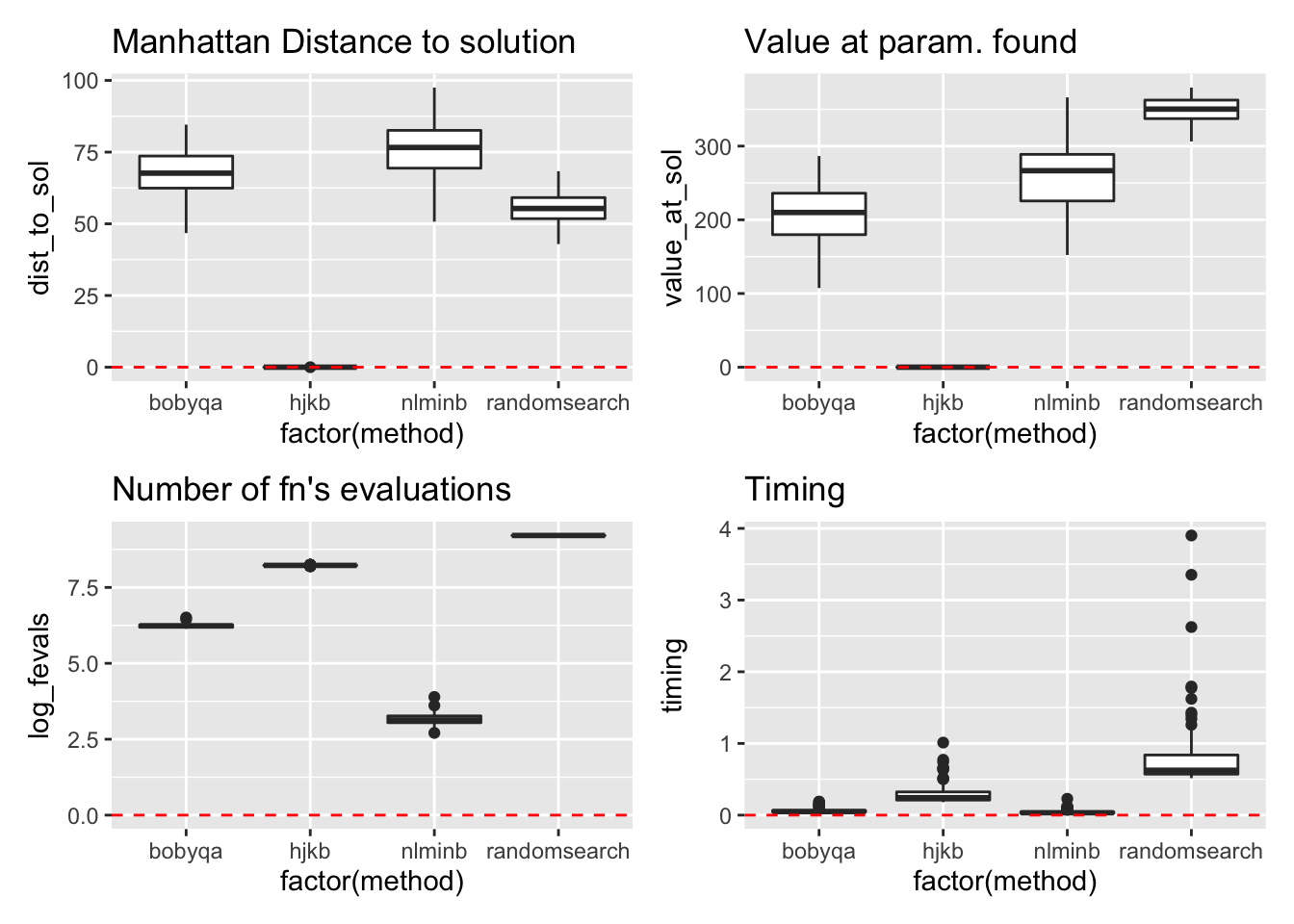Summary for $$f_{10}$$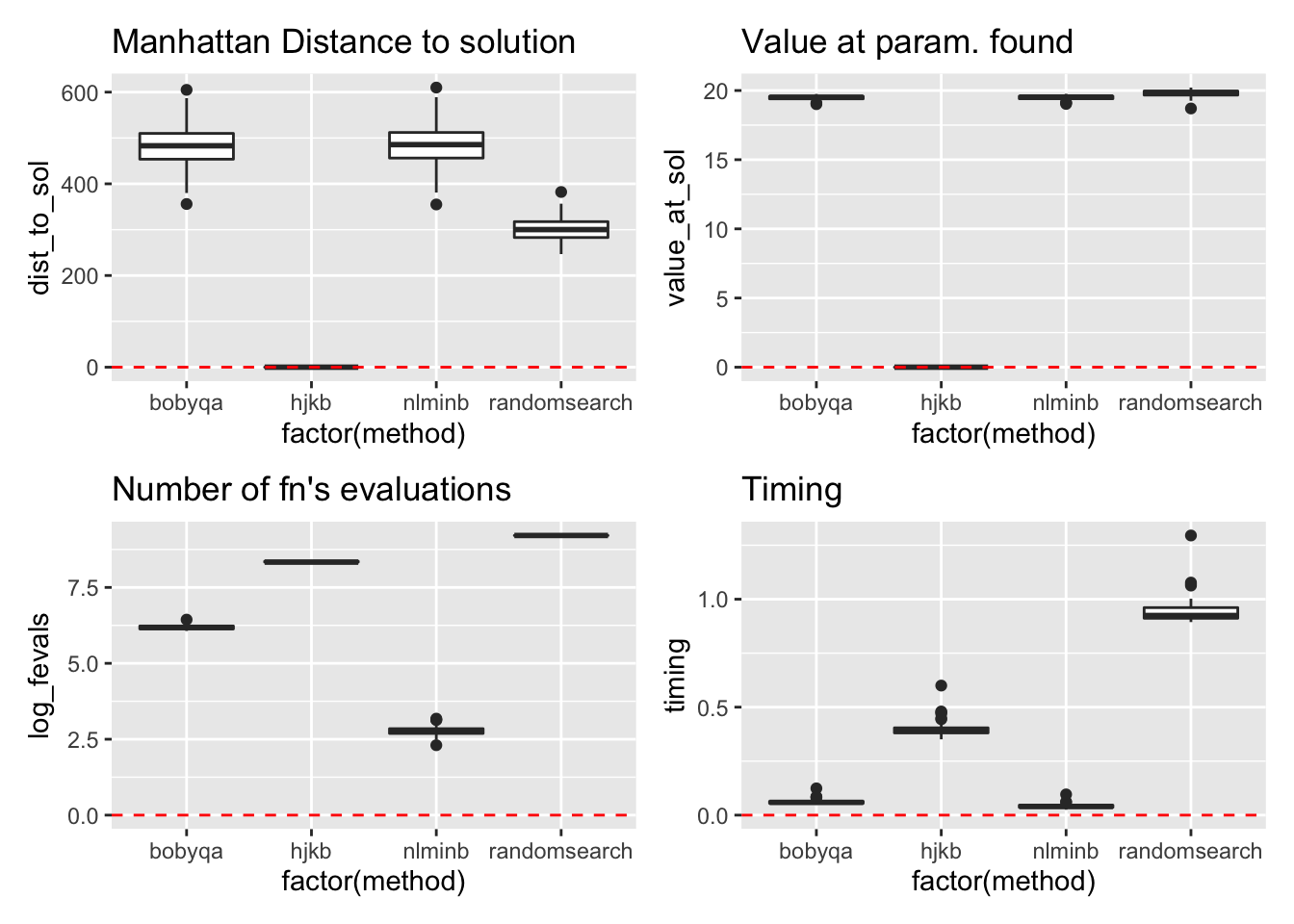Summary for $$f_{11}$$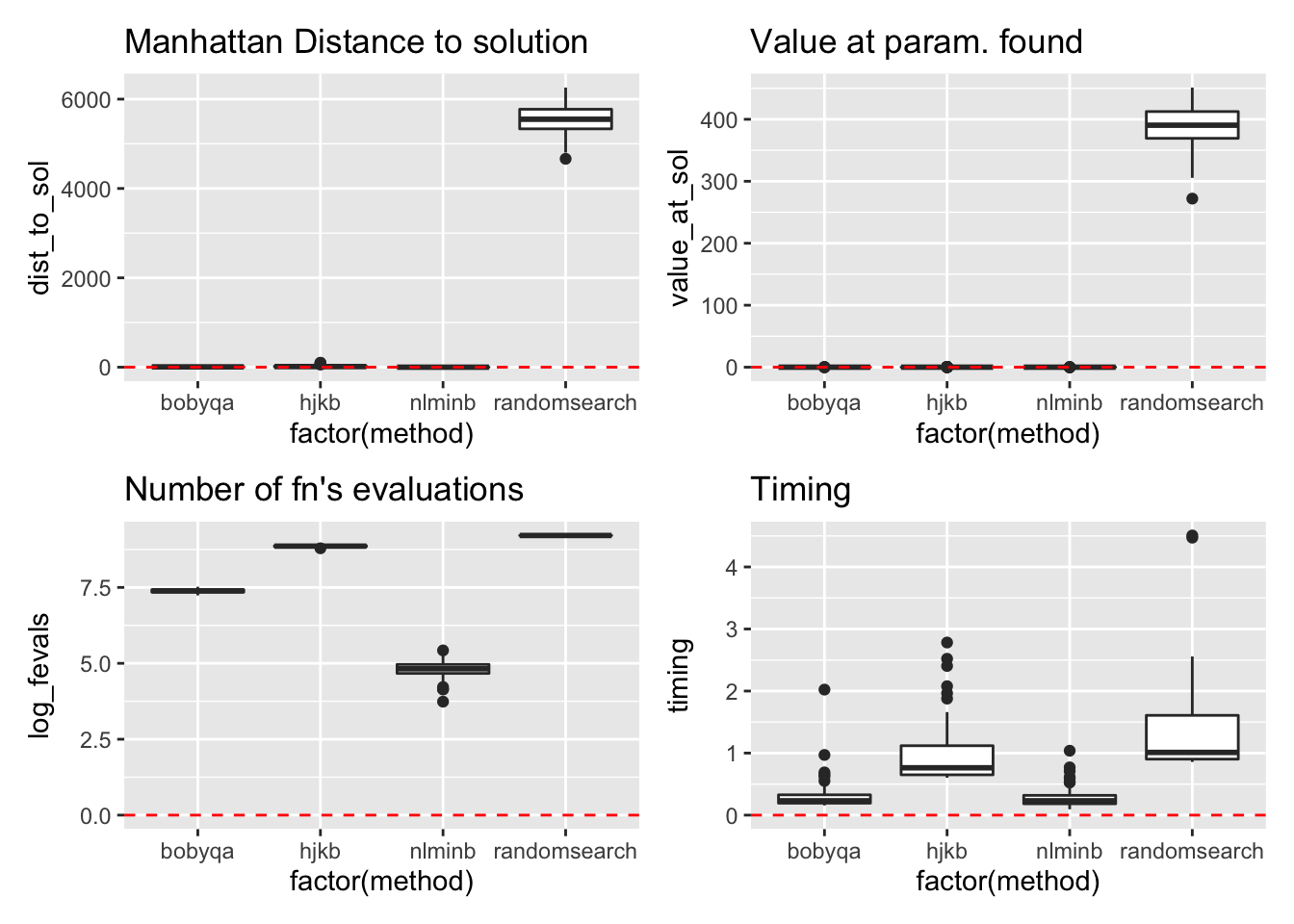The clear winner here, for finding good solutions is the Hooke-Jeeves derivative-free minimization algorithm (hjkb, see , P.296). With that said, hjkb is relatively slow in this setting. It’s 7 times slower than nlminb, if we consider the median timing in seconds. This relative slowness makes hjkb almost unusable on high-dimensional input data.

Conversely, nlminb is the fastest among the 4 chosen optimization methods. In addition, nlminb has the lowest median number of objective functions evaluations. However, nlminb doesn’t find solutions which are close or equal to global minima as consistently as hjkb does. Judging by the first results presented in July 21st’s post (all based on stats::nlminb for computing weights and biases) though, not finding a global minimum doesn’t seem to be hurting BCNs’ ability to generalize – i.e their ability to obtain good accuracy on unseen data.

 Yao, X., Liu, Y., & Lin, G. (1999). Evolutionary programming made faster. IEEE Transactions on Evolutionary computation, 3(2), 82-102.

 Mebane Jr, W. R., & Sekhon, J. S. (2011). Genetic optimization using derivatives: the rgenoud package for R. Journal of Statistical Software, 42, 1-26.

 Quarteroni, Sacco, and Saleri (2007), Numerical Mathematics, Springer.